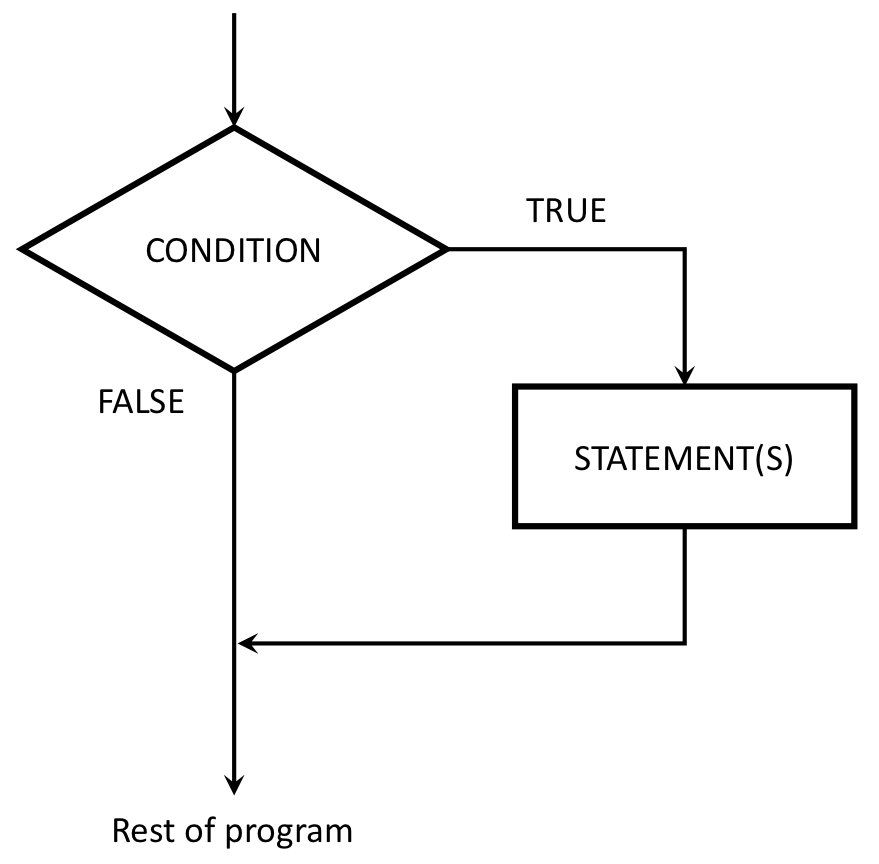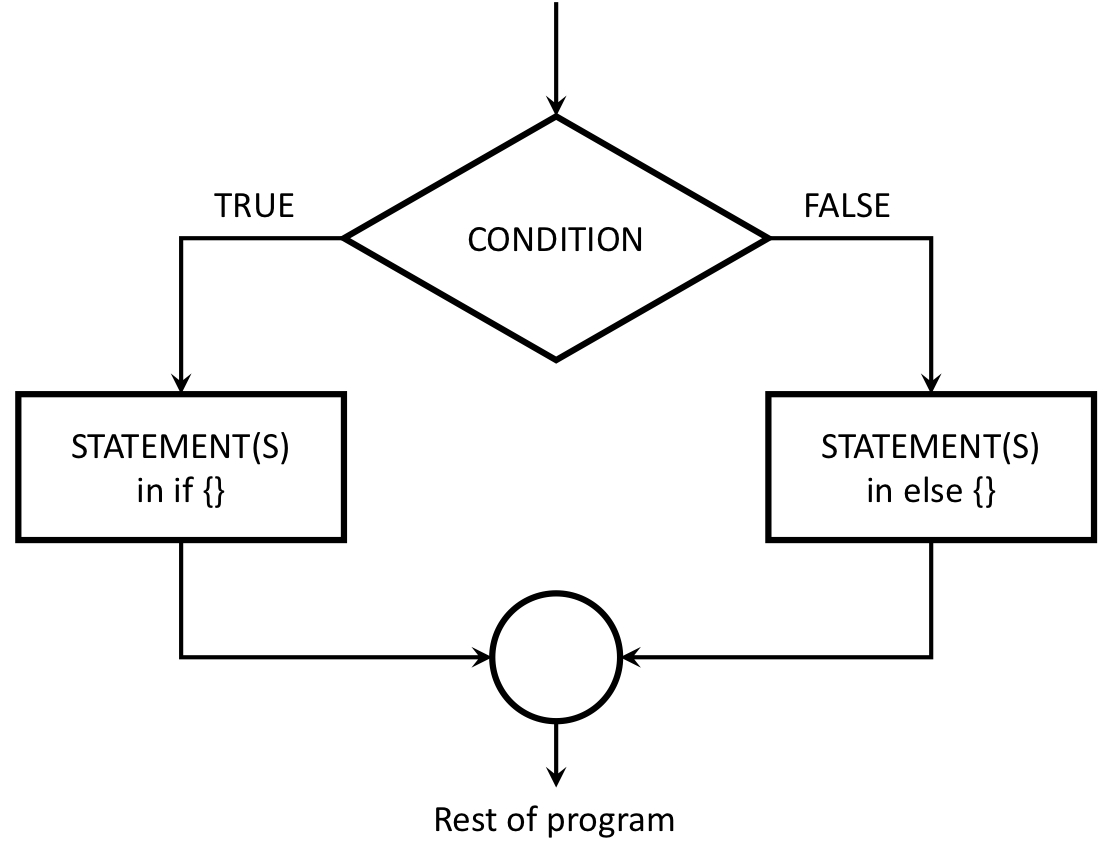### Learning Objectives

• Understand how to use if and else statements to handle conditional programming.

Like most programming languages, R can evaluate conditional statements. A conditional statement is a switch - it tells the code which command to execute depending on a condition that is specified by the programmer.

The most prominent examples of a conditional statement is the if statement, and the accompanying else statement.

# if

The basic format of an if statement in R is as follows:

if ( CONDITION ) {
STATEMENT1
STATEMENT2
ETC
}

If the condition is TRUE, then R will execute the statements contained in the curly braces, otherwise it will skip it. This schematic illustrates the idea:## Example 1

f <- function(x) {
cat("A")
if (x == 0) {
cat("B")
cat("C")
}
cat("D")
}
f(0)
## ABCD
f(1)
## AD

## Example 2

Consider a simple absolute value function. Since abs() is a built-in function, we’ll call ours absValue():

absValue <- function(x) {
if (x < 0) {
x = -1*x
}
return(x)
}
absValue(7)  # Returns 7
##  7
absValue(-7) # Also returns 7
##  7

# if else

You can extend the if statement to include an else statement as well, leading to the following syntax:

if ( CONDITION ) {
STATEMENT1
STATEMENT2
ETC
} else {
STATEMENT3
STATEMENT4
ETC
}

The interpretation of this version is similar. If the condition is TRUE, then the contents of the first block of code are executed; but if it is FALSE, then the contents of the second block of code are executed instead. The schematic illustration of an if-else construction looks like this:## Example

f <- function(x) {
cat("A")
if (x == 0) {
cat("B")
cat("C")
}
else {
cat("D")
if (x == 1) {
cat("E")
} else {
cat("F")
}
}
cat("G")
}
f(0)
## ABCG
f(1)
## ADEG
f(2)
## ADFG

# else if

You can also chain multiple else if statements together for a more complex conditional statement. For example, if you’re trying to assign letter grades to a numeric test score, you can use a series of else if statements to search for the bracket the score lies in:

getLetterGrade <- function(score) {
if (score >= 90) {
} else if (score >= 80) {
} else if (score >= 70) {
} else if (score >= 60) {
} else {
}
}
cat("103 -->", getLetterGrade(103))
## 103 --> A
cat(" 88 -->", getLetterGrade(88))
##  88 --> B
cat(" 70 -->", getLetterGrade(70))
##  70 --> C
cat(" 61 -->", getLetterGrade(61))
##  61 --> D
cat(" 22 -->", getLetterGrade(22))
##  22 --> F

Page sources:

Some content on this page has been modified from other courses, including:

EMSE 4574: Programming for Analytics (Fall 2020) |
Tuesdays | 12:45 - 3:15 PM | Dr. John Paul Helveston | jph@gwu.edu
Content 2020 John Paul Helveston. See the licensing page for details.